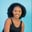Related Tags

operators
dart
communitycreator

# What are operators in Dart programming?Maria Elijah

Operators are special symbols that perform operations on operands. It is used in an expression. An expression consists of operands (called data) and an operator which evaluates to a value.

In Dart, there are a number of built-in operators that may be used to perform various tasks.

### Types of operators

1. Arithmetic Operators
2. Assignment Operators
3. Relational Operators
4. Logical Operators
5. Conditional Operators
6. Type Test Operators
7. Bitwise Operators

#### Arithmetic operators

This class of operators includes:

 Operators Description + Add (a + b) - Subtract (a - b) -expr Unary Minus ( use to reverse the sign of the expression) * Multiply (a * b) / Divide (a / b) ~/ Divides but return an integer result % Modulus ( outputs remainder of two operands) ++ Increment -- Decrement

void main()
{
int x = 14;
int y = 5;
// Using ~/ to divide x and y
var z = x ~/ y;
print("Quotient of x and y: $z"); // Remainder of a and b var d = x % y; print("Remainder of x and y:$d");
}

#### Assignment operators

These operators are used to assign value to operands.

 Operators Description = Equal to ( assign values to the expression) ??= Assignment operator ( assign the value only if it is null )
void main()
{
int x = 14;
int y = 5;

// Assigning value to variable z
var z = x / y;
print(z);

// Assigning value to variable i
var i;  //i has a null value
i ??= x * y; // Value is assign to i as it is null
print(i);
// reassign value to i
i ??= x + y; // Value is not assign because i is not null
print(i);
}

#### Relational operators

These operators perform relational operations on operands, which include:

 Operators Description > Greater than (a > b) < Less than (a < b) >= Greater than or equal to (a >= b) <= Less than or equal to (a <= b) == Equal to (a == b) != Not equal to (a != b)

void main()
{
int x = 14;
int y = 4;

// Greater between x and y
var z = x > y;
print("x is greater than y is $z"); // Equality between x and y var d = x == y; print("x and y are equal is$d");
}

#### Logical operators

These operators are used to combine two or more conditions. A Boolean value is returned by logical operators.

Operators used include:

 Operators Description && And operator ( returns true if both conditions are true) || Or operator ( returns true if one of the conditions is true) ! Not Operator ( reverse the output)
void main()
{
int x = 14;
int y = 5;

// Using And Operator
bool z = x > 5 && y == 5;
print(z);

// Using Or Operator
bool i = x < 20 || y > 15;
print(i);

// Using Not Operator
bool d = !(x > 5);
print(d);
}

#### Conditional operators

These operators perform a comparison on the operands. The operators include:

 Operators Description condition? expr1: expr2 If the condition is true then expr1 is executed else expr2 expr1?? expr2 If expr1 is non-null return its value else return expr2 value
void main()
{
int x = 5;
int y = 7;
// Conditional Statement
var z = (x * y > 30) ? "First expression is printed" : "Second expression is printed";
print(z);

}

#### Type test operators

These operators are used to perform operand comparisons and are useful for checking types at the runtime of a program. Such operators include:

 Operators Description is is (output a Boolean value true if the object has its specific type) is! is not (output a Boolean value false if the object has its specific type)
void main() {
// Type Test Operator
String e  = 'Operators in Dart';
// Using is to compare
print(e is String);
}

#### Bitwise operators

These operators perform bitwise operations on the operands. Below are the operators used:

 Operators Description & Bitwise And (a & b) returns a one in each bit position where both operands' corresponding bits are ones. | Bitwise And (a | b) returns a one for each bit position where the matching bits of either or both operands are ones. ^ Bitwise XOR (a ^ b) returns a one in each bit location when the corresponding bits of either but not both operands are ones. ~ Bitwise NOT( ~a) inverts the operand's bits. << Left shift (a << b) Shifts a in binary representation b (< 32) bits to the left, shifting in zeroes from the right. >> Right shift (a >> b) shifts a in binary representation b (< 32) bits to the right, eliminating bits shifted off.
void main() {
int c = 6;
// Bitwise operator
var bitwise_res =  ~ c;
print("Bitwise result: \$bitwise_res" );
}

These operators allow you to perform a sequence of operations on the same element and also perform multiple methods on the same object.

 Operator Description .. Cascading method (performs multiple methods on the same object)

class EducativeShot {
var title;
var id;
var date;

void set(x, y, z)
{
this.title = x;
this.id = y;
this.date = z;
}

void shot()
{
var myShot = this.title + this.id + this.date;
print(myShot);
}
}

void main()
{
// Creating objects of class EducativeShot
EducativeShot shot1 = new EducativeShot();
EducativeShot shot2 = new EducativeShot();

shot1.set('Operators', ' 0017820', ' 15.10.2021');
shot1.shot();

shot2..set('Operators in Dart programming', ' 0017820', ' 15.10.2021')
..shot();
}

RELATED TAGS

operators
dart
communitycreator

CONTRIBUTORMaria Elijah
RELATED COURSES

View all Courses

Keep Exploring

Learn in-demand tech skills in half the time